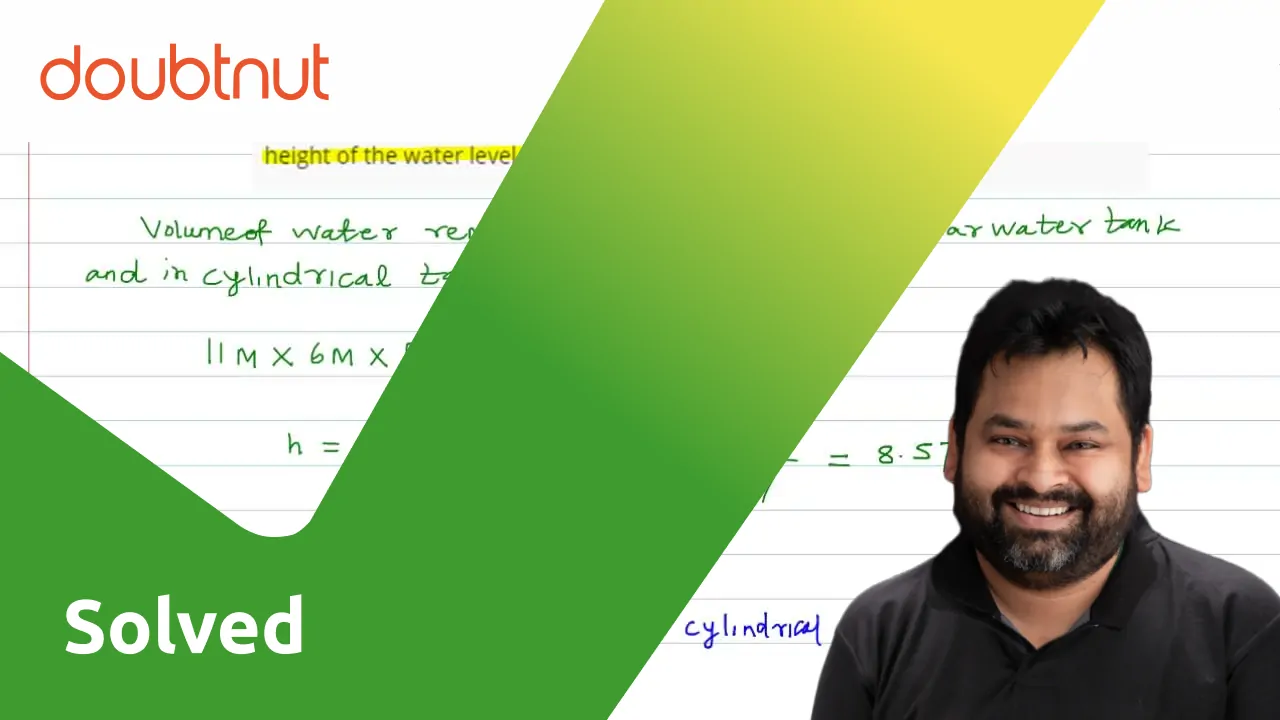Doubtnut App - Get 50 Lakh+ video solutions better than GoogleInstall Now

# A rectangular water tank of base 11m×6m contains water upto a height of 5 m. If the water in the tank is transferred to a cylindrical tank of radius 3.5 m, find the height of the water level in the tank.

Text Solution
Verified by Experts

## Given,dimenison of base of rectaangular tank =11m×6m and height of water g= 5 m Volume of the water in rectangular tank =11×6×5=330m3 Also given radius of the cylindrical tank = 3.5 m Let height of water level in cylinder tank be h. Then, volume of the water is cylindrical =πr2h=π(3.5)2×h =227×3.5×3.5×h According to the question, 330=28.5h [since, volume of water is same in both tanks ] ∴ h=33038.5=3300385 ∴ =8.75mor8.6m Hence, the height of water level in cylindrical tank is 8.6 m.